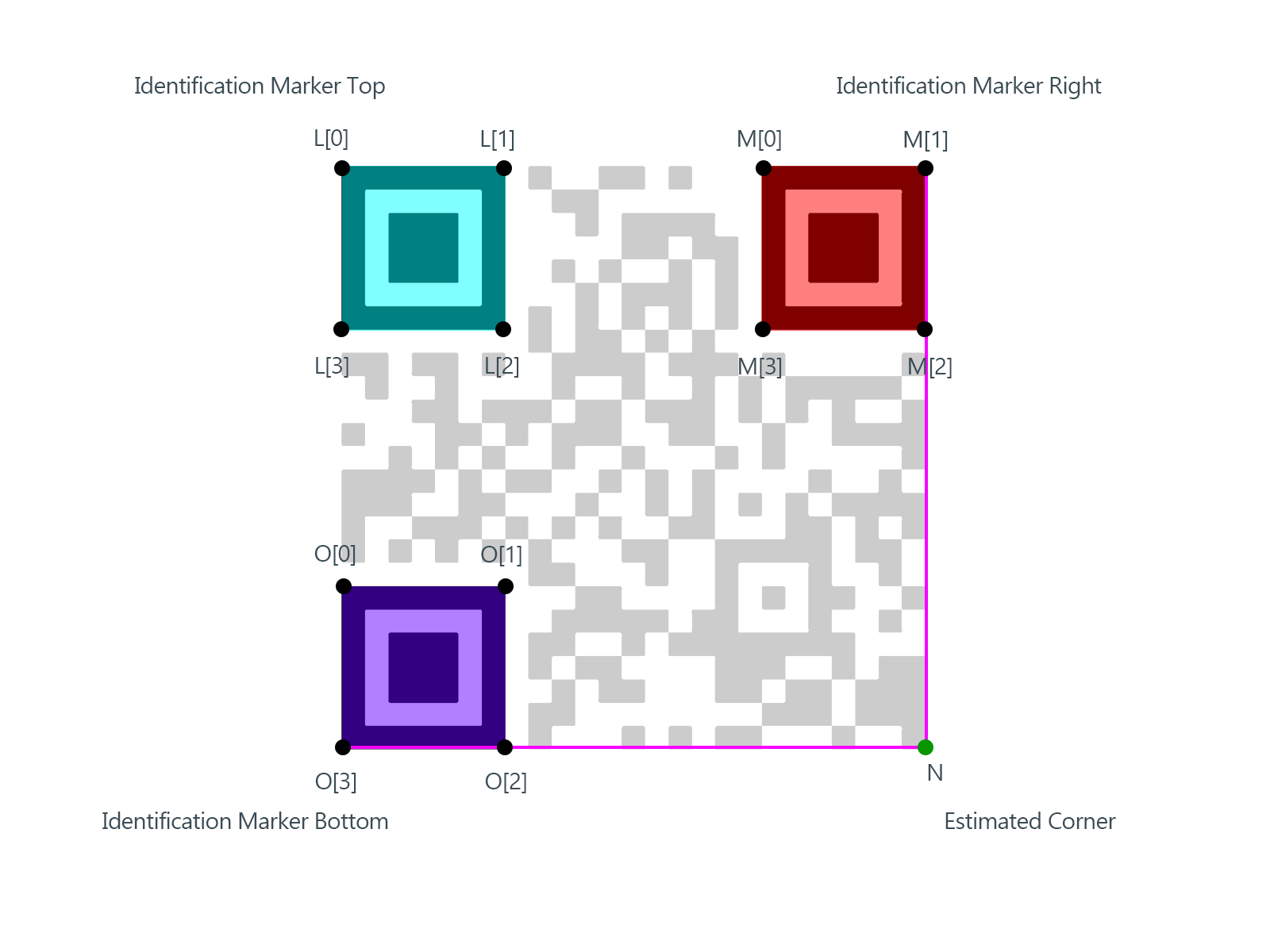## document.write(ssyby);

### OpenCV: QR Code detection and extraction

There are many ways to decode QR Code these days. Here is an attempt to detect QR Code in an image to manipulate within OpenCV.

1. Detection of QR Code Identification markers
We start of the topic by discussing something that is not a part of this sample program. If you need to get on with it, you can skip directly to "Step 1".

The first task is to identify reliable patterns in the QR Code as show above. This can be determined using the technique described below.
A hypothetical Ratio Theorem:
Definition: Ratio of the Areas of N number of two dimensional polygons remain invariant to the uniform geometric transformation.

If ar is the area of a polygon r then,
a1 : a2... : aN = R in 2D plane X then,
a’1 : a’2 ... : aN = R for any other 2D plane Y which is geometric transformation of X

Consider the following example marker in 2D plane A, the areas of the polygons a1, a2 and aare in the ratio 7:5:3. Plane B is obtained by perspective transformation of plane A. However the areas of the polygons a'1, a'and a'plane B can be resolved as multiple of 7, 5 and 3 respectively i.e., 7β:5β:3β or simply 7:5:3. The same is illustrated in the figure below-

Ratio of the areas of the polygons

Proof: I have none. May be some other time.
All great men are gifted with intuition. They know without reasoning or analysis, what they need to know.

This approach is not used in the sample program, instead the structured OpenCV Contour detection technique is used.

Actual Step 1
OpenCV Countour detection does much more then identifying contours in an image. It also stores the relationship and hierarchy amongst the contours. This is nicely explained in the OpenCV documentations and tutorials OpenCV Contour Hierarchy.

Applying contour detection to a QR Code, the contour tracing the perimeter of the pattern polygons has the following characteristics -
It is a contour with subsequent enclosed (nested) contours. Although looking at an Identification marker, you would expect to detect 3 'nested' contours, however the inner boundary is also accounted as a contour resulting in 5 nested contours as shown here.

Nested contours in Identification marker

2. Determining the three distinct Identification markers
Post identification the three markers of the QR Code, the key step now is to determine the orientation of the markers and the positions wrt each other. (i.e., to determine which among the three markers are top, right, bottom  and in what order.)
This is achieved easily using a triangle. The triangle is formed by using the mass centers of each of the three contours as vertices. The Vertex not involved in the largest side is obviously out lying and turns out to be the "top" marker*. Differentiating markers "right" and "bottom" out of the remaining two vertices, requires calculating the slope of the line they form and the position of the marker "top" wrt to this line.

* Limitation: This assumption is not true for acute perspective angles. a different approach is required to resolve and is a limitation of current implementation.

Determination of QR Code Identification Markers

3. Identify the four corners of each Identification markers
In this step we provide the contour points of the polygon to the function which retrieves the four corners of the polygon. The method measures the distance of each point on the perimeter with the center to determine four farthest points. These are likely to be the vertices of the polygon.
This function is implemented and well documented as part of OpenAR. More info openAR.

4. Determining the fourth point of the QR Code
Once four corners of all the three identification markers are obtained it is easy to estimate the fourth corner of the QR Code. The estimation is done by extending the lines forming the boundaries of the other two identification markers namely RIGHT and BOTTOM as shown in the figure below.
The line formed by O and O in BOTTOM identification marker is extended. Similarly line formed by M and M of RIGHT identification marker is extended to meet at N,this estimated position serves as the forth corner of the QR Code.

Reference identification objects in the QR Code5. Orientation and Perspective correction
Having now obtained the 4 corners of the QR Code, namely L, M, N and O, we can apply OpenCV warpPerspective() function to restore the QR Code to readable position.

QR Code Orientation
6. QR Code decoding
QR Code thus recovered from the frame can now be used to extract the data. There are several libraries that are capable and compatible with OpenCV such as ZBar.
Note: This step is not part of the sample.

Another useful library libdecoderqr that was demonstrated in earlier posts, can also be explored. Link

Source:
Git: https://github.com/bharathp666/opencv_qr.git

References:
OpenCV 2D Image co-ordinate system - Link

Equation of a line - Link
Equation of a line with 2 known points -Link
Solutions to equation of a line - Link
Position of a point with respect to a straight line - Link
Perpendicular distance of point from a line formed by two other points - Link
Slope of a line  - Link

1.image.cpp:6:10: fatal error: opencv2/opencv.hpp: No such file or directory
how to solve this?

1.This comment has been removed by the author.

2.install the opencv library

2.Amazing Article thank you so much for the sharing.
Facial Extraction Singapore